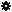﻿ Object Model: PolygonMesh.ConstructPointLocators

PolygonMesh.ConstructPointLocators

## PolygonMesh.ConstructPointLocatorsv5.0

### Description

Builds a PointLocatorData from PolygonMesh-specific topological information. Polygon indices, vertex indices and subtriangle barycentric weights (normalized and positive) are required in order to define each point locator. The vertex indices of a subtriangle must all be part of the corresponding input polygon. The triangle vertex and weight arrays should contain three values for each input polygon.

In order to have more predictable results, it is recommended to specify the subtriangles of vertices corresponding to the actual triangulation of the polygons. Actual polygon triangulation can be retrieved with PolygonFace.TriangleSubIndexArray.

Notice that the returned point locators can be evaluated on any PolygonMesh instance having the same topology.

### C# Syntax

 ```PointLocatorData PolygonMesh.ConstructPointLocators( Object in_pPolygonIndices, Object in_pSubTriangleVertexIndices, Object in_pSubTriangleWeights ); ```

### Scripting Syntax

 ```oReturn = PolygonMesh.ConstructPointLocators( PolygonIndexArray, SubTriangleVertexIndexArray, SubTriangleWeightArray ); ```

### Parameters

Parameter Type Description
PolygonIndexArray 1-dimensional Array An array of polygon indices on which the point locators will be created.
SubTriangleVertexIndexArray Array Can either be a 1-dimensional array of packed triplets, or a 2-dimensional array of triplets. The vertex index triplets define the polygon subtriangle on which the point locators will be created. Vertices of each triplet must be adjacent to the corresponding input polygon.
SubTriangleWeightArray Array An array of Double, either formatted as a 1D array of packed barycentric coordinate triplets, or as a 2D array of barycentric coordinate triplets. In order to have proper results, the sum of the weights of each triplet must be 1.0, and each weight should be a positive value.

### Examples

#### JScript Example

 ```// // This example creates point locators at random surface locations, // and positions a Null at each of these point locators. // // Even if these point locators are simply randomly constructed, // this example shows how to do it in the most accurate way, // which is to specify subtriangles which correspond to the actual // polygon triangulation. // NewScene( null, false ); var SphereGeom = CreatePrim("Sphere", "MeshSurface").ActivePrimitive.Geometry; var NbPtLocators = 50; var PolygonIndexArray = new Array(NbPtLocators); var SubTriangleVertexArray = new Array(NbPtLocators*3); var SubTriangleWeightArray = new Array(NbPtLocators*3); var Polygons = SphereGeom.Polygons; for(i = 0; i < NbPtLocators; i++) { PolygonIndexArray[i] = Math.floor(Math.random() * 0.999999 * Polygons.Count); var Polygon = Polygons.Item(PolygonIndexArray[i]); var SubTri = Math.floor(Math.random() * 0.999999 * (Polygon.NbPoints-2)); var PolygonVertices = Polygon.Vertices; var TriangleSubIndices = Polygon.TriangleSubIndexArray.toArray(); SubTriangleVertexArray[i*3] = PolygonVertices.Item(TriangleSubIndices[SubTri*3]).Index; SubTriangleVertexArray[i*3+1] = PolygonVertices.Item(TriangleSubIndices[SubTri*3+1]).Index; SubTriangleVertexArray[i*3+2] = PolygonVertices.Item(TriangleSubIndices[SubTri*3+2]).Index; var W1 = Math.random(); var W2 = Math.random(); var W3 = Math.random(); var WSum = W1+W2+W3; SubTriangleWeightArray[i*3] = W1/WSum; SubTriangleWeightArray[i*3+1] = W2/WSum; SubTriangleWeightArray[i*3+2] = W3/WSum; } var RandomPointLocators = SphereGeom.ConstructPointLocators(PolygonIndexArray, SubTriangleVertexArray, SubTriangleWeightArray); CreateNullsAtPointLocations(SphereGeom, RandomPointLocators); function CreateNullsAtPointLocations( InGeom, InPointLocators ) { var SpherePositions = InGeom.EvaluatePositions(InPointLocators).toArray(); var SphereNormals = InGeom.EvaluateNormals(InPointLocators).toArray(); var TempVector = XSIMath.CreateVector3(); var TempRotation = XSIMath.CreateRotation(); for (i = 0; i < SpherePositions.length; i+=3) { var NullObj = GetPrim( "Null" ); TempVector.Set(SpherePositions[i], SpherePositions[i+1], SpherePositions[i+2]); NullObj.LocalTranslation = TempVector; TempVector.Set(SphereNormals[i], SphereNormals[i+1], SphereNormals[i+2]); TempRotation.SetFromXYZAxes( TempVector, TempVector, TempVector ); NullObj.LocalRotation = TempRotation; } } ```